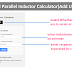Here, in this Inductor Calculator, you can calculate the equivalent inductance of a circuit that contains multiple Inductors. Here, you can calculate inductance for both types of circuits whether the inductors are connected in series or parallel.

Series and Parallel Inductor Calculator

## Series and Parallel Inductor Calculator

### Parallel Inductor Calculation

Use these short forms for different units:

Henry (H), Millihenry (mH), Microhenry (uH), Nanohenry (nH), and Picohenry (pH)

### How to Use This Calculator?

To use the above inductor calculator, follow these steps,

1. Select Calculation Mode: The calculator offers two modes: Series and Parallel. So first of all, choose the appropriate mode based on the type of inductor calculation you need.

2. Enter Inductor Values: After selecting the mode you need to put the inductance values in the input field labeled "Enter inductor values (comma-separated)." Enter the values of the inductors you want to calculate. Separate multiple values with commas. For example, if you have four inductors with values 10, 4.7, 11, and 2.9, you would enter 10,4.7,11,2.9.

3. Enter Respective Units: Now you need to enter the respective units for the values you have entered. For this, you should enter units in the input field labeled "Enter respective units (comma-separated)." Enter the units corresponding to each inductor value. The units should be provided in the same order as the values entered in the previous step. Use the short forms for different units: H (Henry), mH (Millihenry), uH (Microhenry), nH (Nanohenry), and pH (Picohenry). Separate the units with commas. For example, if the units for the previous example are H, mH, uH, and nH, you would enter H,mH, uH,nH.

4. Calculate: Click the "Calculate" button to perform the calculation based on the entered values and units. The calculator will display the result below the button, indicating the calculated inductance value.

5. Reset: If you want to perform another calculation, you can click the "Reset" button to clear the input fields and start afresh.

6. Switch Calculation Modes: If you again need to switch between Series and Parallel modes, simply click the corresponding tab button ("Series" or "Parallel") to open the desired calculator.

Make sure to provide the values and units accurately to obtain the correct results.

### Formula

Here are the formulas, the calculator uses for the calculation.

Series Inductance

When inductors are connected in series, the total inductance is given by the sum of individual inductances.

Ltotal = L1 + L2 + L3 + ... + Ln

where Ltotal is the total inductance and L1, L2, L3, ..., Ln are the individual inductances of the series-connected inductors.

Parallel Inductance

When inductors are connected in parallel, the total inductance is given by the reciprocal of the sum of the reciprocals of individual inductances.

1 / Ltotal = 1 / L1 + 1 / L2 + 1 / L3 + ... + 1 / Ln

To calculate the parallel inductance, take the reciprocal of both sides:

Ltotal = 1 / (1 / L1 + 1 / L2 + 1 / L3 + ... + 1 / Ln)

where Ltotal is the total inductance and L1, L2, L3, ..., Ln are the individual inductances of the parallel-connected inductors.

### Example

Let's take an example to calculate the inductance values by using the above formulas.

Suppose we have three inductors: L1 = 2 mH, L2 = 3 mH, and L3 = 4 mH.

Series Inductance Calculation

To find the total inductance when the inductors are connected in series, we can simply add their individual inductance values as below,

Ltotal = L1 + L2 + L3
= 2 mH + 3 mH + 4 mH
= 9 mH

Therefore, when the inductors are connected in series, the total inductance is 9 mH.

Parallel Inductance Calculation

To find the total inductance when the inductors are connected in parallel, we need to use the reciprocal formula as below,

1 / Ltotal = 1 / L1 + 1 / L2 + 1 / L3
= 1 / 2 mH + 1 / 3 mH + 1 / 4 mH
= (1/2 + 1/3 + 1/4) mH

We take the reciprocal of both sides,

Ltotal = 1 / (1/2 + 1/3 + 1/4) mH

Now if we simplify this expression,

Ltotal = 1 / (6/12 + 4/12 + 3/12) mH
= 1 / (13/12) mH
= 12 / 13 mH = 0.923 mH

Therefore, when the inductors are connected in parallel, the total inductance is 0.923 mH.

Thank you for visiting the Website. Keep visiting for more Updates.

Series and Parallel Inductor Calculator (Add Unlimited)Reviewed by Author on July 26, 2023 Rating: 5